# Energetics Flashcards

Set Details Share
created 11 years ago by hdiwza
5,140 views
updated 11 years ago by hdiwza
Page to share:
Embed this setcancel
COPY
code changes based on your size selection
Size:
X

1

What energy changes occur when chemical bonds are formed and broken?
A. Energy is absorbed when bonds are formed and when they are broken.
B. Energy is released when bonds are formed and when they are broken.
C. Energy is absorbed when bonds are formed and released when they are broken.
D. Energy is released when bonds are formed and absorbed when they are broken.

D

2

The temperature of a 2.0 g sample of aluminium increases from 25°C to 30°C. How many joules of heat energy were added? (Specific heat of Al = 0.90 J g–1K–1)
A. 0.36
B. 2.3
C. 9.0
D. 11

C

3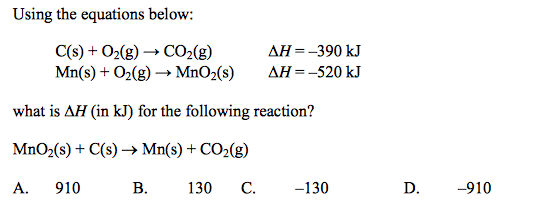B

4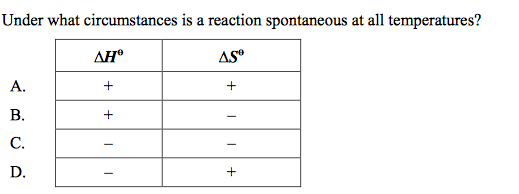D

5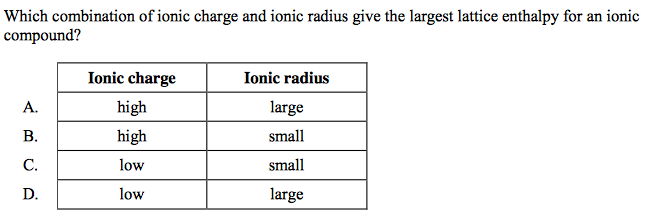B

6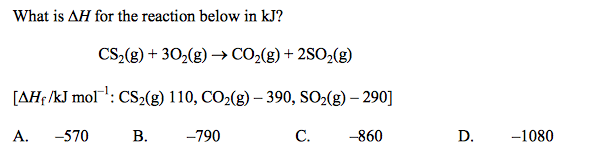D

7

Which statements about exothermic reactions are correct?
I. They have negative DH values
II. The products have a lower enthalpy than the reactants.
III. The products are more energetically stable than the reactants.
A. I and II only
B. I and III only
C. II and III only
D. I, II and III

D

8

A sample of a metal is heated. Which of the following are needed to calculate the heat absorbed by the sample?
I. The mass of the sample
II. The density of the sample
III. The specific heat capacity of the sample
A. I and II only
B. I and III only
C. II and III only
D. I, II and III

B

9

The average bond enthalpies for O—O and O==O are 146 and 496 kJ mol–1 respectively. What is the enthalpy change, in kJ, for the reaction below?
H—O—O—H(g) -> H—O—H(g) + 1⁄2O==O(g)
A. – 102
B. + 102
C. + 350
D. + 394

A

10

Which reaction has the greatest positive entropy change?
A. CH4(g) + 11⁄2O2(g) → CO(g) + 2H2O(g)
B. CH4(g) + 11⁄2O2(g) → CO(g) + 2H2O(l)
C. CH4(g) + 2O2(g) → CO2(g) + 2H2O(g)
D. CH4(g) + 2O2(g) → CO2(g) + 2H2O(l)

A

11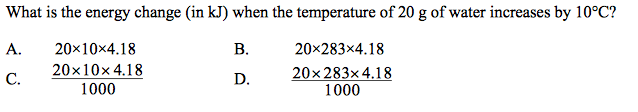C

12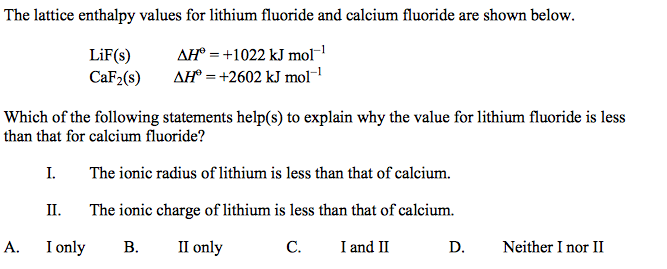B

13

When the solids Ba(OH)2 and NH4SCN are mixed, a solution is produced and the temperature drops.
Ba(OH)2(s) + 2NH4SCN(s) → Ba(SCN)2(aq) + 2NH3(g) + 2H2O(l)
Which statement about the energetics of this reaction is correct?
A. The reaction is endothermic and DH is negative.
B. The reaction is endothermic and DH is positive.
C. The reaction is exothermic and DH is negative.
D. The reaction is exothermic and DH is positive.

B

14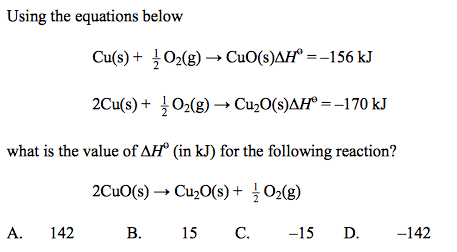A

15

Which reaction occurs with the largest increase in entropy?
A. Pb(NO3)2(s) + 2KI(s) → PbI2(s) + 2KNO3(s)
B. CaCO3(s) → CaO(s) + CO2(g)
C. 3H2(g) + N2(g) → 2NH3(g)
D. H2(g) + I2(g) → 2HI(g)

B

16

The ∆H and ∆S values for a certain reaction are both positive. Which statement is correct about the spontaneity of this reaction at different temperatures?
A. It will be spontaneous at all temperatures.
B. It will be spontaneous at high temperatures but not at low temperatures.
C. It will be spontaneous at low temperatures but not at high temperatures.
D. It will not be spontaneous at any temperature

B

17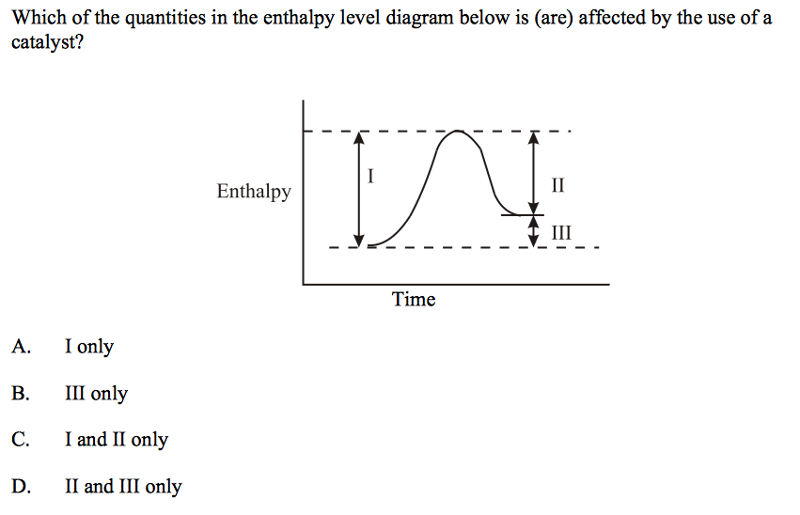C

18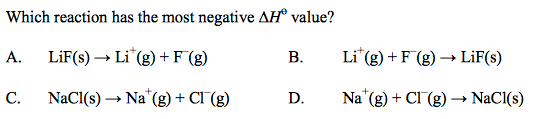B

19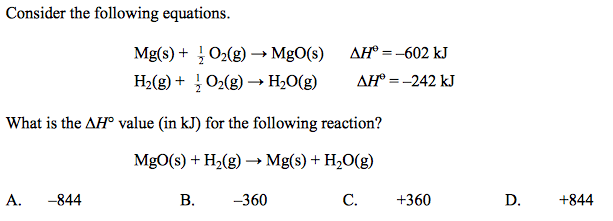C

20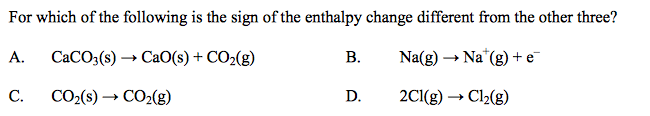D

21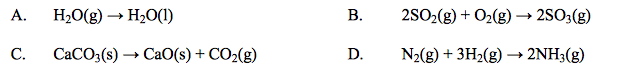Which reaction has a positive entropy change, ∆S?

C

22

Separate solutions of HCl(aq) and H2SO4(aq) of the same concentration and same volume were completely neutralized by NaOH(aq). X kJ and Y kJ of heat were evolved respectively. Which statement is correct?
A. X = Y
B. Y = 2X
C. X = 2Y
D. Y = 3X

B

23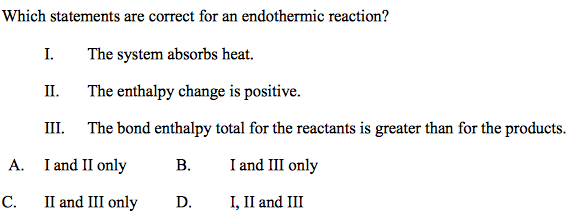A

24

The mass m (in g) of a substance of specific heat capacity c (in J g–1 K–1 ) increases by t°C. What is the heat change in J?
A. mct
B. mc(t + 273)
C. mct
D. mc(t + 273)/1000

A

25

The average bond enthalpy for the C―H bond is 412 kJ mol–1. Which process has an enthalpy change closest to this value?
A. CH4(g) → C(s) + 2H2(g)
B. CH4(g) → C(g) + 2H2(g)
C. CH4(g) → C(s) + 4H(g)
D. CH4(g) → CH3(g) + H(g)

D

26

For a certain reaction at 298 K the values of both ∆H and ∆S are negative. Which statement about the sign of ∆G for this reaction must be correct?
A. It is negative at all temperatures.
B. It is positive at all temperatures.
C. It is negative at high temperatures and positive at low temperatures.
D. It cannot be determined without knowing the temperature.

D

27

Which type of reaction is referred to in the definition of standard enthalpy change of formation?
A. the formation of a compound from its elements
B. the formation of a crystal from its ions
C. the formation of a molecule from its atoms
D. the formation of a compound from other compounds

A

28

The following equation shows the formation of magnesium oxide from magnesium metal.
2Mg(s) + O2(g)->2MgO(s) DHӨ = –1204kJ Which statement is correct for this reaction?
A. 1204 kJ of energy are released for every mol of magnesium reacted.
B. 602 kJ of energy are absorbed for every mol of magnesium oxide formed.
C. 602 kJ of energy are released for every mol of oxygen gas reacted.
D. 1204 kJ of energy are released for every two mol of magnesium oxide formed.

D

29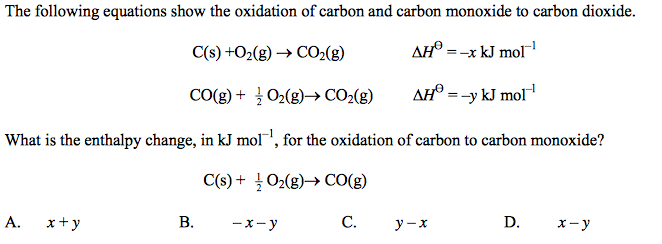C

30

A simple calorimeter was used to determine the enthalpy of combustion of ethanol. The experimental value obtained was –920 kJ mol–1. The Data Booklet value is –1371 kJ mol–1. Which of the following best explains the difference between the two values?
A. incomplete combustion of the fuel
B. heat loss to the surroundings
C. poor ventilation in the laboratory
D. inaccurate temperature measurements

B

31

What is the correct order of decreasing entropy for a pure substance? A. gas A. gas > liquid > solid
B. solid > liquid > gas
C. solid > gas > liquid
D. liquid > solid > gas

A

32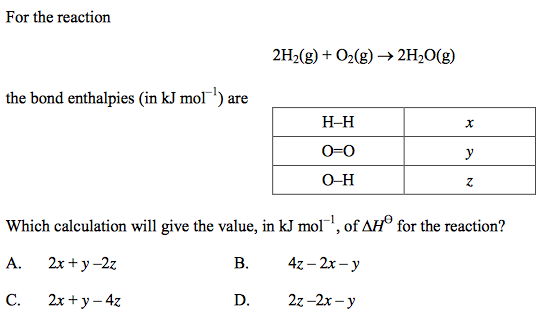C

33

Which statement about bond enthalpies is correct?
A. Bond enthalpies have positive values for strong bonds and negative values for weak
bonds.
B. Bond enthalpy values are greater for ionic bonds than for covalent bonds.
C. Bond breaking is endothermic and bond making is exothermic.
D. The carbon–carbon bond enthalpy values are the same in ethane and ethene.

C

34

An equation for a reaction in which hydrogen is formed is
CH4 + H2O -> 3H2 + CO DHӨ = +210 kJ
Which energy change occurs when 1 mol of hydrogen is formed in this reaction?
A. 70 kJ of energy are absorbed from the surroundings.
B. 70 kJ of energy are released to the surroundings.
C. 210 kJ of energy are absorbed from the surroundings.
D. 210 kJ of energy are released to the surroundings.

A

35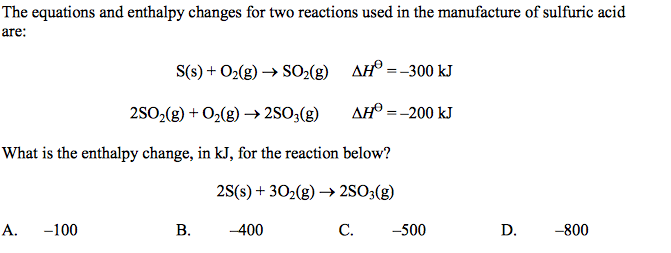D

36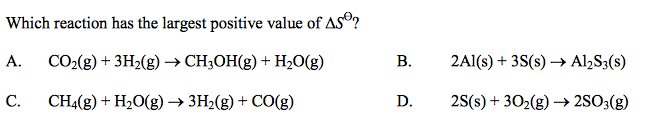C

37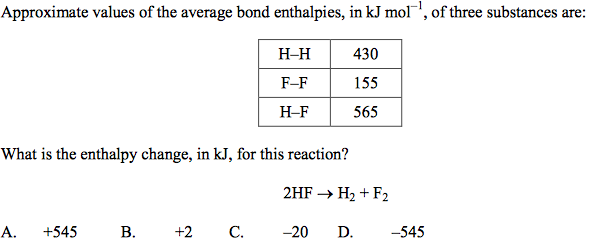A

38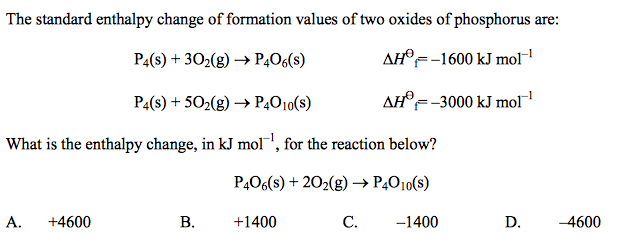C

39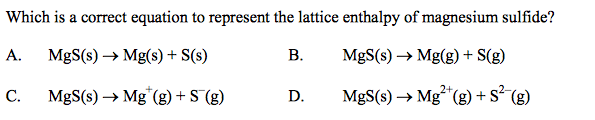D

40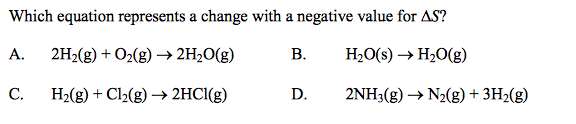A

41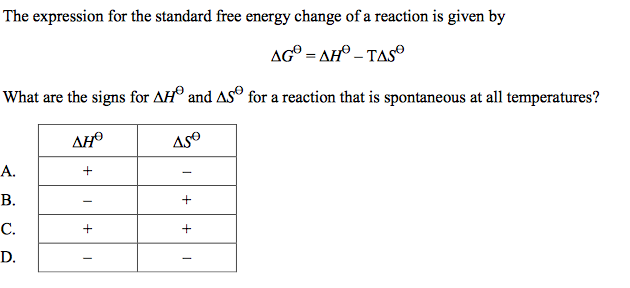B

42

Which statement is correct for an endothermic reaction?
A. The products are more stable than the reactants and DH is positive.
B. The products are less stable than the reactants and DH is negative.
C. The reactants are more stable than the products and DH is positive.
D. The reactants are less stable than the products and DH is negative.

C

43

Which statement is correct about the reaction shown?
2SO2(g) + O2(g) <-> 2SO3(g) DH = –196 kJ
A. 196 kJ of energy are released for every mole of SO2(g) reacted.
B. 196 kJ of energy are absorbed for every mole of SO2(g) reacted.
C. 98 kJ of energy are released for every mole of SO2(g) reacted.
D. 98 kJ of energy are absorbed for every mole of SO2(g) reacted.

C

44

Which statements are correct for all exothermic reactions?
I. The enthalpy of the products is less than the enthalpy of the reactants.
II. The sign of H is negative.
III. The reaction is rapid at room temperature.
A. I and II only
B. I and III only
C. II and III only
D. I, II, and III

A

45

Which are characteristics of ions in an ionic compound with a large lattice enthalpy value?
A. Large ionic radius and high ionic charge
B. Small ionic radius and low ionic charge
C. Large ionic radius and low ionic charge
D. Small ionic radius and high ionic charge

D

46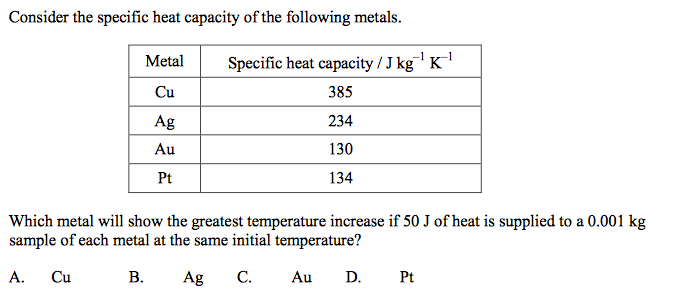C

47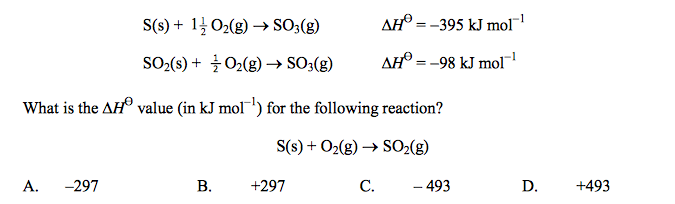Consider the following reactions:

A

48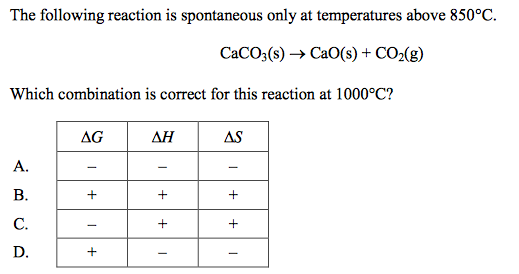C

49

Which statement is correct for an endothermic reaction?
A. Bonds in the products are stronger than the bonds in the reactants.
B. Bonds in the reactants are stronger than the bonds in the products.
C. The enthalpy of the products is less than that of the reactants.
D. The reaction is spontaneous at low temperatures but becomes non-spontaneous at high temperatures.

B

50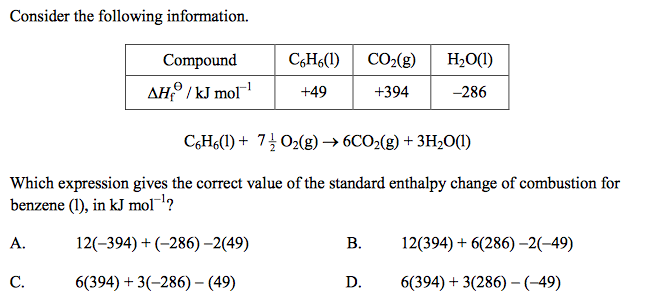C

51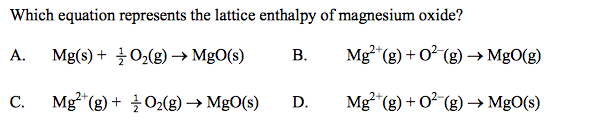D

52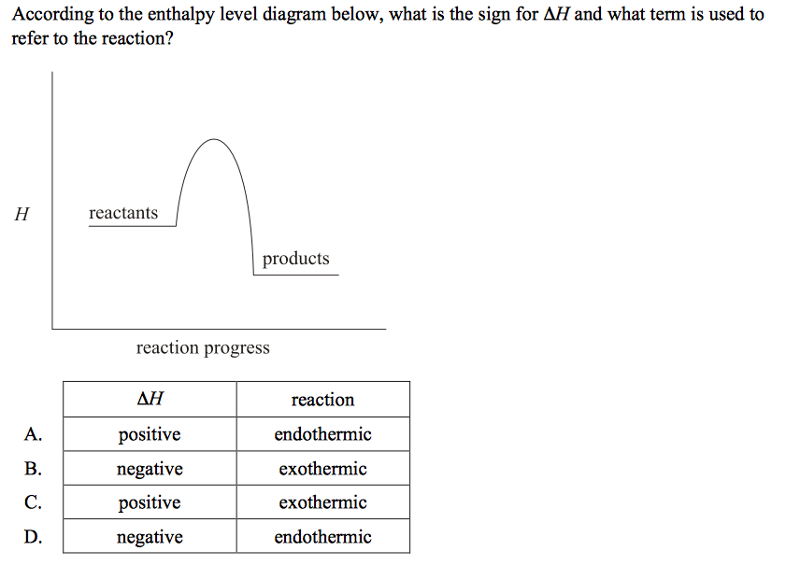B

53

When 40 joules of heat are added to a sample of solid H2O at –16.0°C the temperature increases to –8.0°C. What is the mass of the solid H2O sample? [Specific heat capacity of H2O(s) = 2.0 J g–1K–1]
A. 2.5 g
B. 5.0 g
C. 10 g
D. 160 g

A

54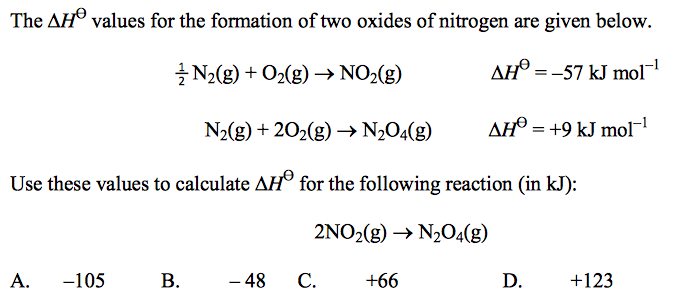D

55

The HӨ and SӨ values for a reaction are both negative. What will happen to the spontaneity of this reaction as the temperature is increased?
A. The reaction will become more spontaneous as the temperature is increased.
B. The reaction will become less spontaneous as the temperature is increased.
C. The reaction will remain spontaneous at all temperatures.
D. The reaction will remain non-spontaneous at any temperature.

B

56

How much energy, in joules, is required to increase the temperature of 2.0 g of aluminium from 25 to 30°C? (Specific heat of Al = 0.90 J g–1 K–1).
A. 0.36
B. 4.5
C. 9.0
D. 54

C

57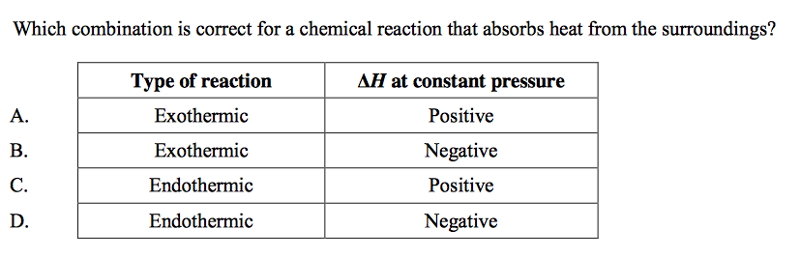C

58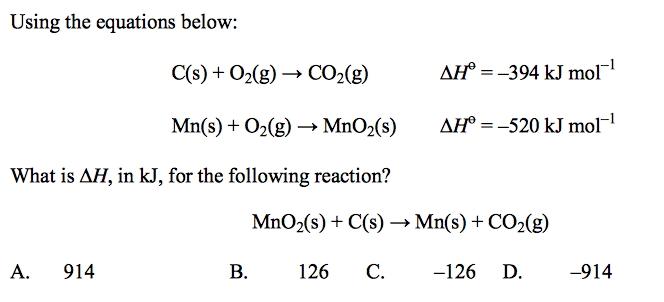B

59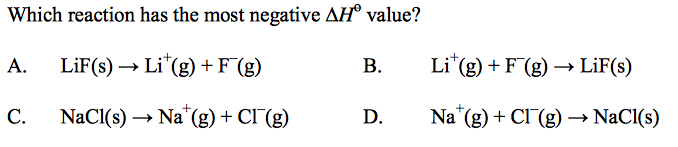B

60C

61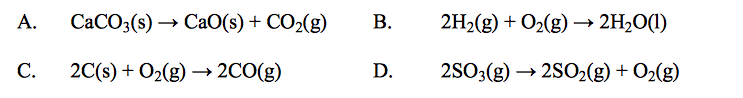Which reaction causes a decrease in the entropy of the system?

B

62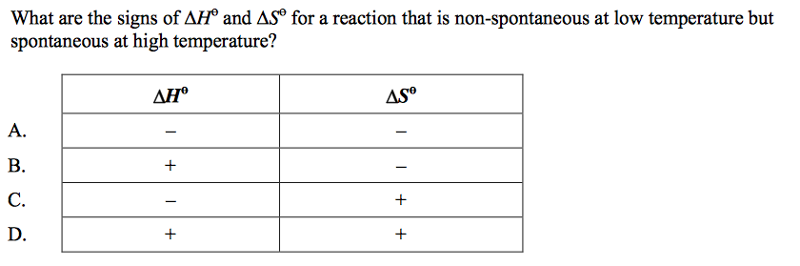D